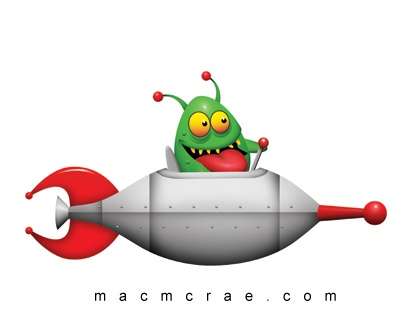Alien ProjectileTwo projectiles, one fired from the surface of the earth with speed 5 m/s and the other fired from the surface of a planet with initial speed 3 m/s, trace identical trajectories. Neglecting friction effect the value of acceleration due to gravity on the planet is? Assume $g = 9.8 \text{ ms}^{-2}$.

×